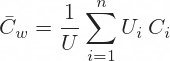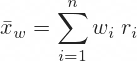# Weighted Average Method (Cost Accounting)

## Weighted Average Method Logic

The weighted average method is one of the most common methods of inventory and cost accounting. It is also known as Average Cost Method or AVCO. It is an alternative to FIFO (first in first out) and LIFO (last in first out), which are other commonly used cost accounting methods.

The core idea of the weighted average method is to assume that all units that are currently in the inventory have been purchased at the same price (unit cost).

## Weighted Average Method Formula for Unit Cost

Average unit cost under the weighted average method is calculated as weighted average of unit costs of past purchases, according to the following formula:... where there have been n purchases of inventory (i = 1, 2, 3, ..., n) and:

• Ui is the number of units purchased on the i-th inventory purchase
• Ci is the unit cost on the i-th inventory purchase
• U is the total number of units purchased

The weighted average unit cost is simply the total amount that has been paid for the inventory divided by the number of units in the inventory.

The formula is of course the same as the general weighted average formula:... where:

• wi is the weight of item i.
• xi is the value of item i.

## Weighted Average Method Example

There is a small refinery. It buys crude oil and uses it to produce gasoline, diesel, and other oil products.

• In March it buys 10,000 barrels of oil at 85 USD/barrel (total cost of this purchase is USD 850,000).
• In June it buys 7,000 barrels at 100 USD/barrel (total cost is USD 700,000).
• In August is buys 8,000 barrels at 80 USD/barrel (total cost is USD 640,000).
• At the end of the accounting period, it has used 22,000 barrels of crude oil in production. 3,000 barrels remain in inventory.

What has been the cost for the accounting period under the weighted average method?

### Average Unit Cost

Total cost for all inventory is the sum of the costs of individual purchases:

10,000 x 85 + 7,000 x 100 + 8,000 x 80 =

= 850,000 + 700,000 + 640,000 =

= USD 2,190,000 for all 25,000 barrels.

Unit cost under the weighted average method is simply the total cost divided by number of units:

2,190,000 / 25,000 = 87.60 USD/barrel

### Cost for the Period and Ending Inventory

Out of the total 25,000 barrels, only 22,000 have been used in production and 3,000 remain as inventory for the following accounting period.

The cost in the current accounting period is the average unit cost times number of units used:

87.60 x 22,000 = USD 1,927,200

Ending inventory is the average unit cost times number of units remaining:

87.60 x 3,000 = USD 262,800

... which must be the same as the total cost of all purchases less the cost of the units used in production:

2,190,000 – 1,927,000 = USD 262,800

## When to Use Weighted Average Method

The weighted average method is typically used when inventory items are commodities or close to commodities and it is difficult to tell which particular item was purchased when, and therefore difficult to assign specific unit cost. It requires less sophisticated inventory management and accounting than other methods like FIFO or LIFO.

By remaining on this website or using its content, you confirm that you have read and agree with the Terms of Use Agreement.

We are not liable for any damages resulting from using this website. Any information may be inaccurate or incomplete. See full Limitation of Liability.

Content may include affiliate links, which means we may earn commission if you buy on the linked website. See full Affiliate and Referral Disclosure.

We use cookies and similar technology to improve user experience and analyze traffic. See full Cookie Policy.